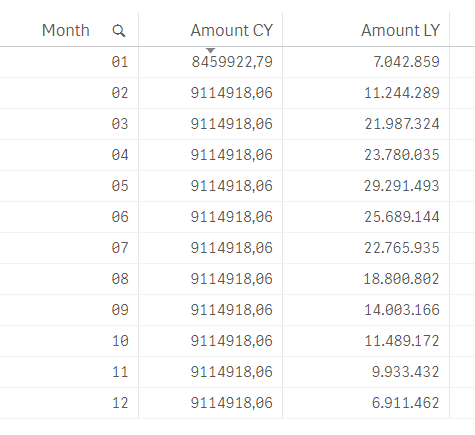Announcements
Product Release Webinar: Qlik Insider airing December 6! REGISTER TODAY!
cancel
Showing results for
Did you mean:Partner - Contributor III

## Accumulative sum till current month

Hi all,

I'm having some trouble figuring the following out.

I have a accumulation per month of sum(amount) for the current year and previous year. But my line chart for the current year should stop at the current month.

I've used the following formula for the calculation of the accumulation:

rangesum(above(Sum({<Year={\$(=max(Year))}>}Amount),0,rowno()))

The calculation works just fine. See the results in the table below:As a result my linechart looks like this:But my client wants the Amount CY line to stop at month 02.

Is there a way to get this done?

1 Solution

Accepted SolutionsChampion III

=if(Month <= Month(today()) , rangesum(above(Sum({<Year={\$(=max(Year))}>}Amount),0,rowno())))

Vineeth Pujari
If a post helps to resolve your issue, please accept it as a Solution.
3 RepliesChampion III

=if(Month <= Month(today()) , rangesum(above(Sum({<Year={\$(=max(Year))}>}Amount),0,rowno())))

Vineeth Pujari
If a post helps to resolve your issue, please accept it as a Solution.Champion III

or give this a try

=rangesum(above(Sum({<Year={\$(=max(Year))} , Month = {"<=\$(=Month(Today()))"}>}Amount),0,rowno())))

Vineeth Pujari
If a post helps to resolve your issue, please accept it as a Solution.Partner - Contributor III
Author

Thank you so much Vineeth!

I tried several things but I must have missed this one.Community Browser